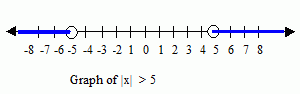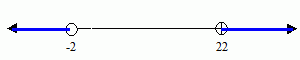Email us to get an instant 20% discount on highly effective K-12 Math & English kwizNET Programs!

#### Online Quiz (WorksheetABCD)

Questions Per Quiz = 2 4 6 8 10

### High School Mathematics10.2 Solve Absolute Value Inequalities

 The equation |x| = 5 means that the distance between x and 0 is 5. The solutions of the equation are 5 and -5 because they are the only numbers whose distance from 0 is 5. The inequality |x| < 3 means that the distance between x and 0 is less than 3, and The inequality |x| > 3 means that the distance between x and 0 is greater than 3.Solving Absolute Value Inequality: The inequality |ax+b| < c where c > 0 is equivalent to the compound inequality -c < ax+b < c The inequality |ax+b| > c where c > 0 is equivalent to the compound inequality ax+b < -c or ax + b > c In the equalities above, < can be replaced by Ł and > can be replaced by ł. Examples: Solve |x| ł 8 The solutions are x ł 8 and x Ł -8Solve |x| Ł 0.7 |x| Ł 0.7 -------- original equation Rewrite as two equations x Ł 0.7 or x ł -0.7 -0.7Ł x Ł 0.7Solve |-4x-5|+3 < 9 |-4x-5|+3 < 9 subtracting 3 both sides of the inequation |-4x-5|+3 -3 < 9-3 |-4x-5| < 6 -6 < -4x-5 < 6 adding 5 to the inequations -6+5 < -4x-5+5 < 6+5 -1 < -4x < 11 Dividing by -4 reverses the inequality sign 0.25 > x > -2.75 This can also be written as -2.75 < x < 0.25Solve |10 - x| > 12 10 - x > 12 or 10 - x < -12 10 -x > 12 subtracting 10 both sides 10 -x-10 > 12-10 -x > 2 Multiplying both sides by (-1) changes the sign x < -2 10 - x < -12 subtracting 10 both sides 10 -x-10 < -12-10 -x < -22 Multiplying both sides by (-1) changes the sign x > 22 Therefore x < -2 or x > 22|x + 2| < -1 Since |x + 2| cannot be negative, |x + 2| cannot be less than -1. So, the solution set is the empty set. Solution = { } |2y -1| ł -4 Since |2y - 1| is always greater than or equal to 0, the solution set is {y|y is a real number} The graph is the entire number line. Directions: Solve the following questions. Also write at least 5 examples of your own. Solve them and graph the solution. Click here for additional questions less than or equal to Ł greater than or equal to ł
 Q 1: |14 - m| > 6m > 8 or m >20m < 8 or m < 20m > 8 or m >20m < 8 or m > 20 Q 2: If |z| Ł 1/4. Then z Ł _____ or z ł _______ -1/4, 1/41/4 , -1/4 Q 3: |x| < 4-4 < x < 4-4 > x > 0-4 > x < 4-4 < x > 4 Q 4: The inequality 1/6|2x - 1| + 2 ł 5 the solution set is {x: x Ł _____ or x ł ____} -15/2, 9/25/3, -7/5-17/2, 19/2 Q 5: |-4x-5| + 3 < 9-2.75 < x < 0.25-2 < x < 5-2.5 < x < 2.50 < x < 2 Q 6: Solve the inequality |-2x + 1| > 2 {x: x > -1/2 or x > 3/2}{x: x < -1 or x > 1/2}{x: x < -3/2 or x > 1/2}{x: x < -1/2 or x > 3/2} Q 7: |d+4| ł 3. Then d ł -1 or d Ł -7TrueFalse Q 8: Solve the inequality |2x - 1| < 9.{x: -4 < x > 5}{x: -4 < x < 5}{x: -4 > x > 5}{x: -4 > x < 5} Question 9: This question is available to subscribers only! Question 10: This question is available to subscribers only!

#### Subscription to kwizNET Learning System offers the following benefits:

• Unrestricted access to grade appropriate lessons, quizzes, & printable worksheets
• Instant scoring of online quizzes
• Progress tracking and award certificates to keep your student motivated
• Unlimited practice with auto-generated 'WIZ MATH' quizzes